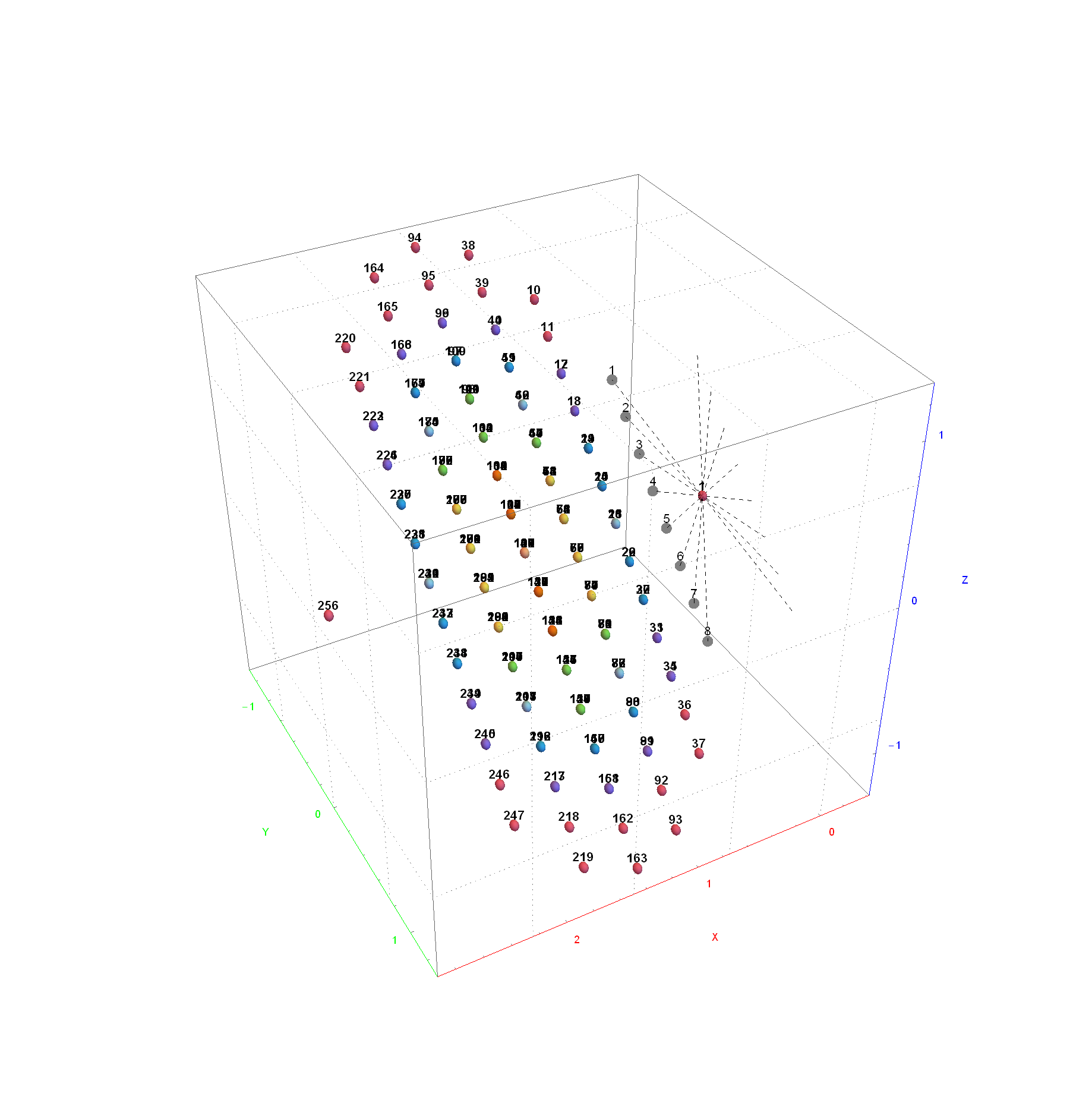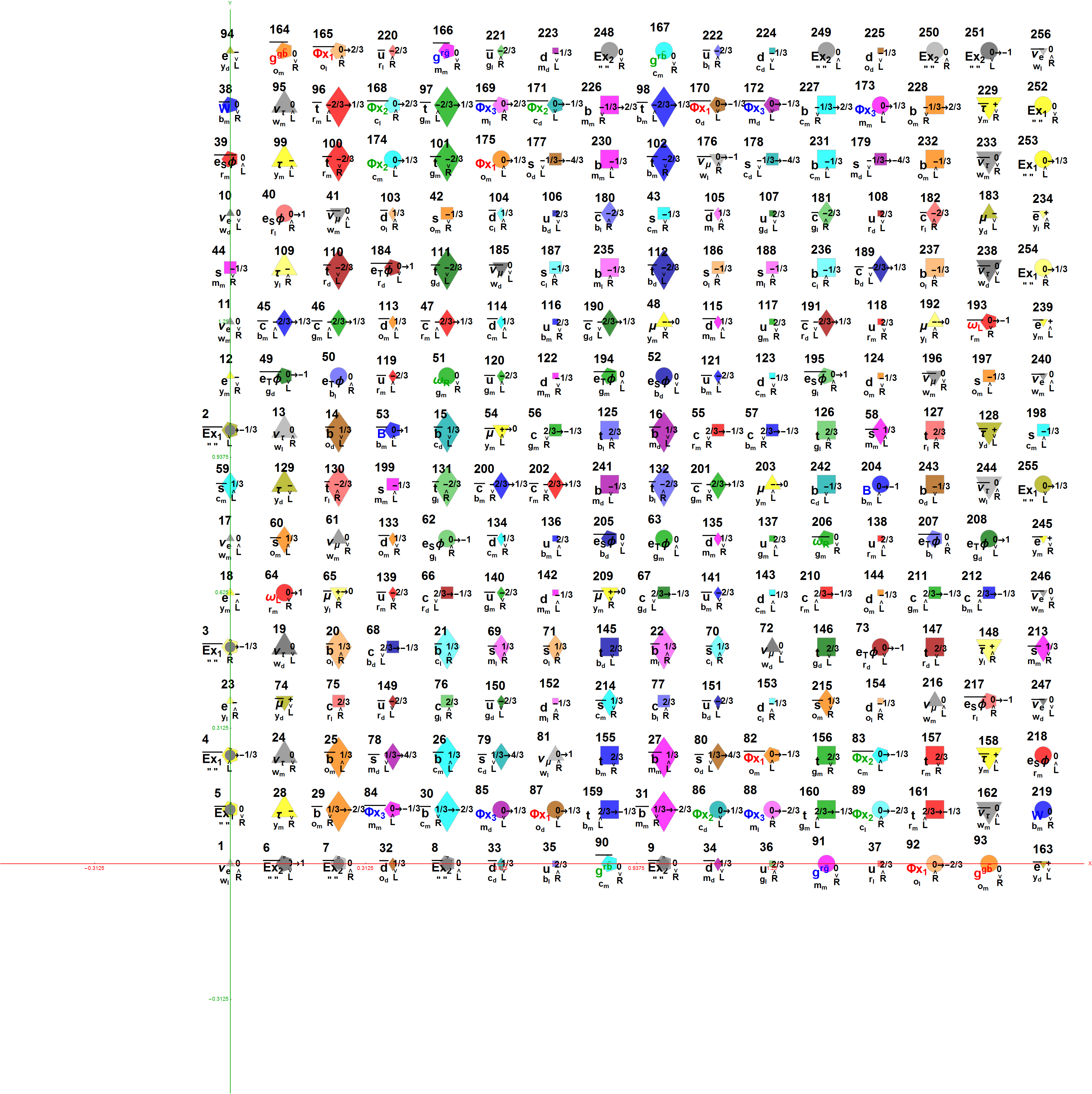# A 3D projection of E8 (binary coordinates) to Pascal triangle related columns

This uses “binary” vertices of E8 (all 0’s or 1’s) from the Pascal triangle / Clifford algebra construction.
Notice the 8 excluded “generator” vertices are the axis 1-8, which show the projection orientation {X,Y,Z} vectorx. These sometimes are added to the 240 vertex “dimension count” of E8. There are a number of overlaps in the vertices in some columns, which are indicated by the color of the vertex.Just for good measure, here is a 2D 16×16 matrix projection of the same “binary” E8 vertices. It shows the particle physics label with the E8 vertex number.